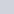# Implicit Cost calculation

• This topic has 1 reply, 2 voices, and was last updated 2 years, 4 months ago byLynn Roden.
• Creator
Topic
• #129276Yahia
Participant

Dears
There is something not clear to me when calculate the implicit cost.
In the example in the video when Mr. Hock calculate the economic depreciation for the implicit costs he subtract the hole economic depreciation value .
I think it should be subtract the difference between the accounting depreciation and the economic depreciation, because in that example Sarah’s economic profit reduce with \$30,000 depreciation expense

Thanks

• This topic was modified 2 years, 4 months ago byYahia.
• This topic was modified 2 months, 3 weeks ago byKevin Hock.
Viewing 1 replies (of 1 total)
• Author
Replies
• #129305Lynn Roden
HOCK international

Hi Yahia,

Please note that the calculation of economic profit does not include the accounting depreciation. The calculation of economic profit includes economic depreciation instead of accounting depreciation. Therefore, there is no need to calculate the difference between the two types of depreciation. Accounting depreciation is used to calculate accounting profit. Economic depreciation is used to calculate economic profit. It is one or the other in both situations.

If you wanted to, you could start your calculation of economic profit with the accounting profit of 75,000. If you were to do that, then yes, you would need to deduct the difference between the two depreciations from the accounting profit because the accounting depreciation is included in the calculation of accounting profit. That would be calculated as follows:

Accounting profit: 75,000

Minus increase in economic depreciation over accounting depreciation: 10,000

Minus lost wages: 40,000

Minus lost interest: 10,000

Minus lost accounting profit: 20,000

Equals economic profit: (5,000)

As you can see, the economic profit is the same as it is calculated in the example: (5,000).

This example is also in your book. I think if you review the example in the book, you will see more clearly what I mean.

Lynn

Viewing 1 replies (of 1 total)
• You must be logged in to reply to this topic.